# Triangle Construction

We all are already aware of the concept of a triangle. A triangle is a three-sided polygon made up of three sides having three angles. It is to be noted that in the construction of a triangle, the three sides and angle may or may not be equal in dimensions.

Practical geometry, which deals with the construction of different geometrical figures, is an important branch of Geometry. Using a set of geometrical tools such as rulers, compasses and protractors, different shapes like squares, triangles, circles, hexagons, etc. can be constructed. The only condition is that you should be aware of the properties of these figures that set them apart from one another. You are already aware of the construction of lines, angles, bisectors, etc. This knowledge is extended to the construction of triangles. But before we move to that, in this section, we bring to you all the properties of triangles that you need to keep in mind before moving over to triangle construction.

## Properties of Triangles

Different triangles have different properties. Some of the common properties of all triangles are listed below.

• The sum of the three interior angles of a triangle is 180°.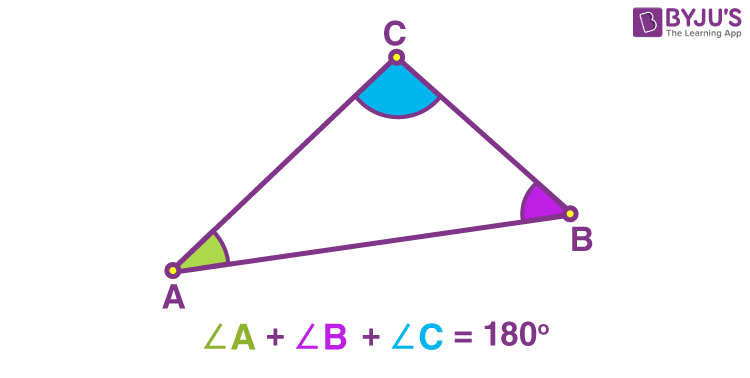• The measure of the exterior angle is equal to the sum of the opposite interior angles.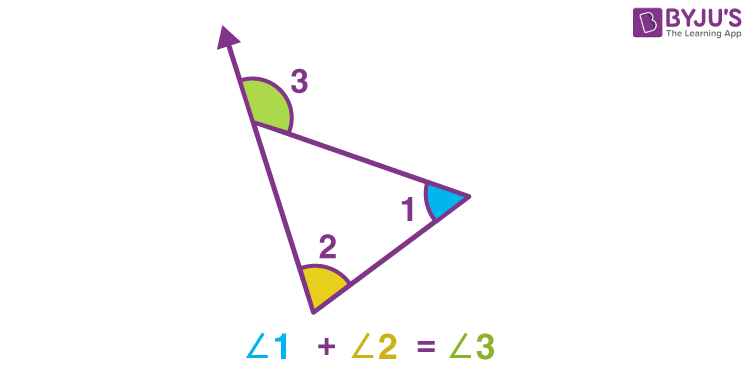• The sum of lengths of two sides of a triangle is always greater than the length of the third side.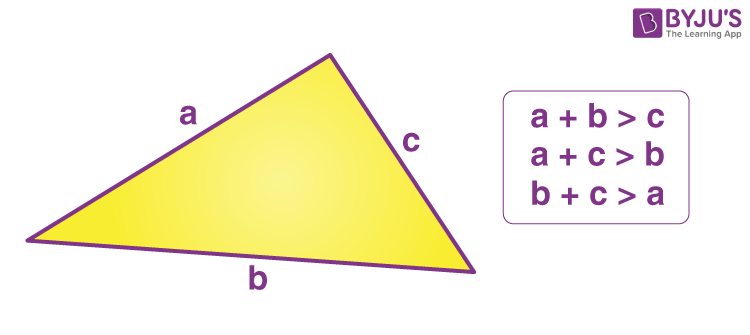• Pythagoras Theorem: In a right-angled triangle, the square of the length of the hypotenuse (the side opposite to the right angle) is equal to the sum of the squares of the length of the other two sides.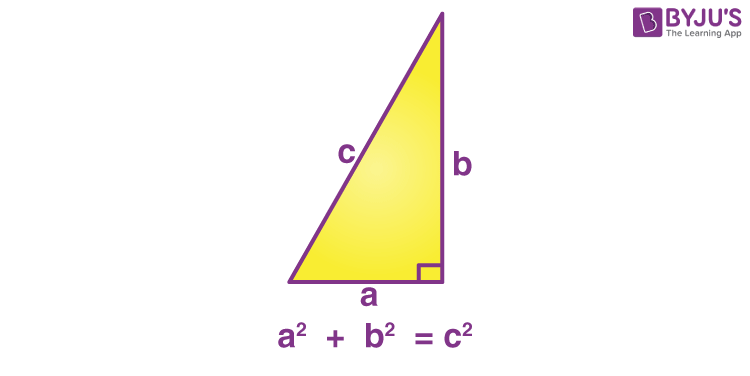## Types of Construction of Triangles

We know that the triangle can be classified based on sides and angles. Similarly, the construction of triangles can also be performed if we know the sides and the measurement of angles. The three types of construction of triangles are:

• Triangle construction Based on SSS Criteria
• Triangle Construction Based  on SAS Criteria
• Triangle Construction Based on ASA Criteria

### Construction of Triangle – SSS Criteria

In the triangle construction based on SSS criteria, the length of all three sides is required.

Example 1:

Construct a triangle ABC whose side lengths are 3 cm, 5 cm and 6 cm.

Construction:

Step 1: Draw the longest side using ruler. (i.e) AB = 6 cm.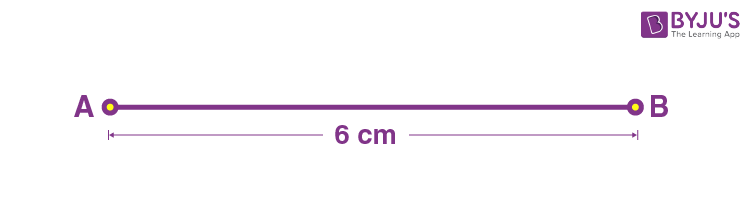Step 2: Take a compass, and draw an arc above line AB from point A, whose measurement is 5 cm.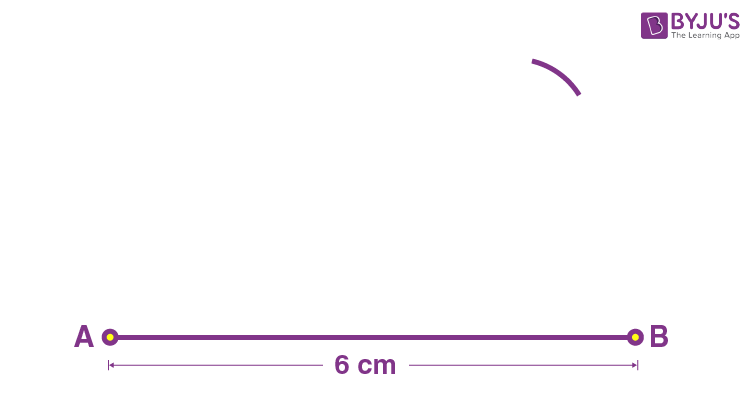Step 3: Similarly from Point B, draw an arc whose measurement is 3 cm. (Note: Draw an arc in such a way that both the arcs intersect at a point)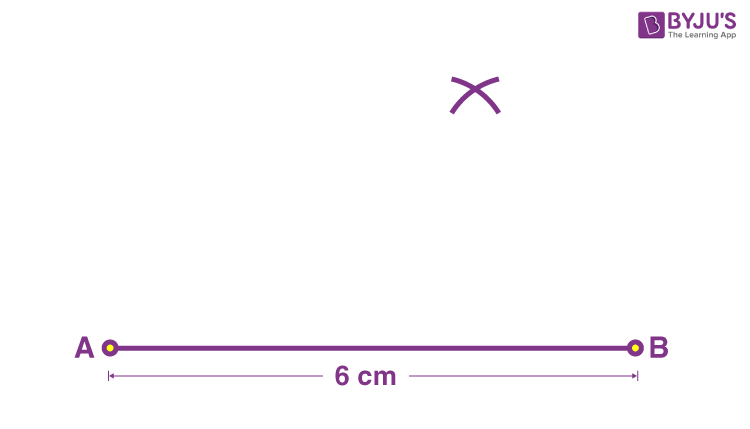Step 4: Mark the intersection point as C and join CA and CB using a ruler.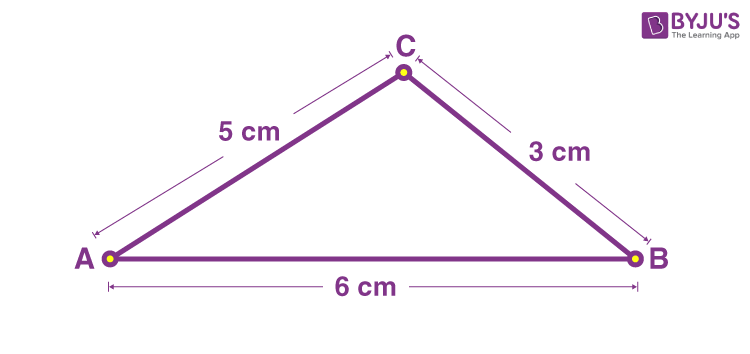Hence, ABC is the required triangle.

### Construction of Triangle – SAS Criteria

In the construction of the SAS triangle, we need to know the side lengths of two sides and the angle between them are required.

Example 2:

Construct a triangle ABC, whose side lengths are 4 cm and 6 cm and the angle between them is 40°.

Construction:

Step 1: Draw the longest side of the triangle using a ruler. (i.e AC = 6 cm)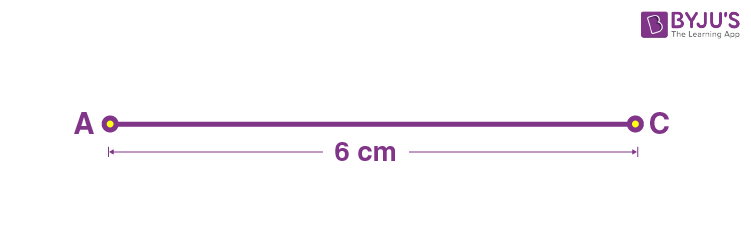Step 2: Place the centre point of the protractor on point A and measure 40°. (i.e) Use inner reading and count 0° from the horizontal line to 40°. Mark this point as B.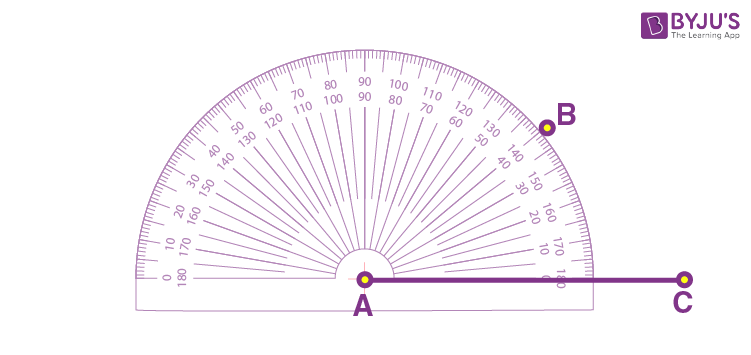Step 3: Using a ruler, draw a line AB, such that AB = 4 cm.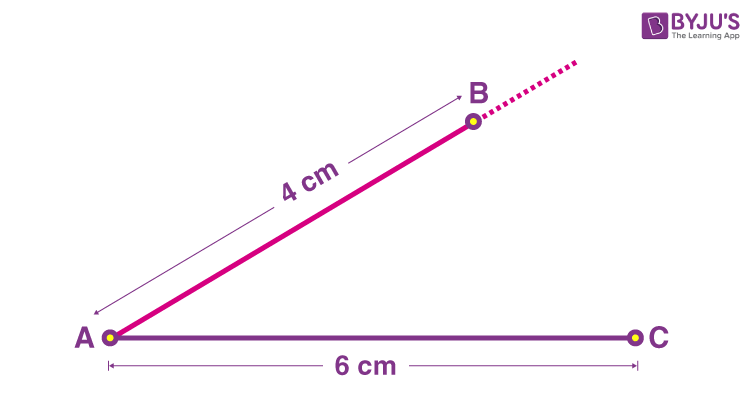Step 4: Now draw the third side of the triangle by joining points B and C.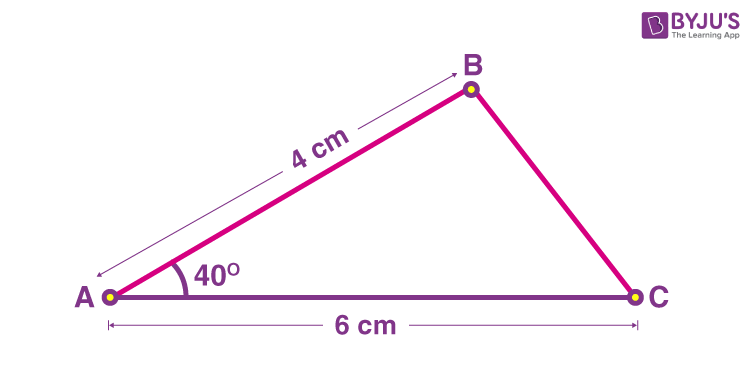Hence, ABC is the required triangle.

### Construction of Triangle – ASA Criteria

In the construction of a triangle based on ASA criteria, we need to know the measurement of two angles and the side length between them is required.

Example 3:

Construct a triangle whose two angle measurements are 40° and 70° and the side length between them is 8 cm.

Construction:

Step 1: Draw the line of length 8 cm using a ruler. (i.e) AB = 8 cm.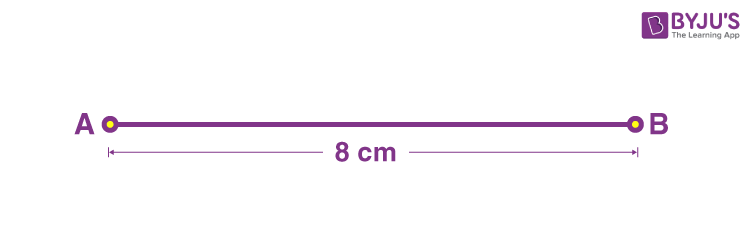Step 2: Place the centre of the protractor on point A and measure 40° [Use the inner reading]. Now, put the construction mark at 40°.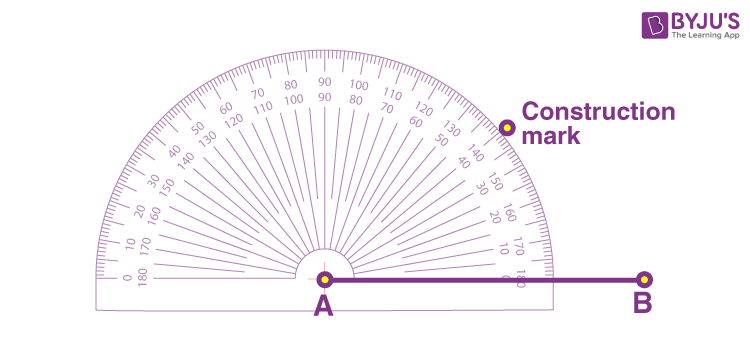Step 3: Using the ruler, draw a long line from A through the construction mark.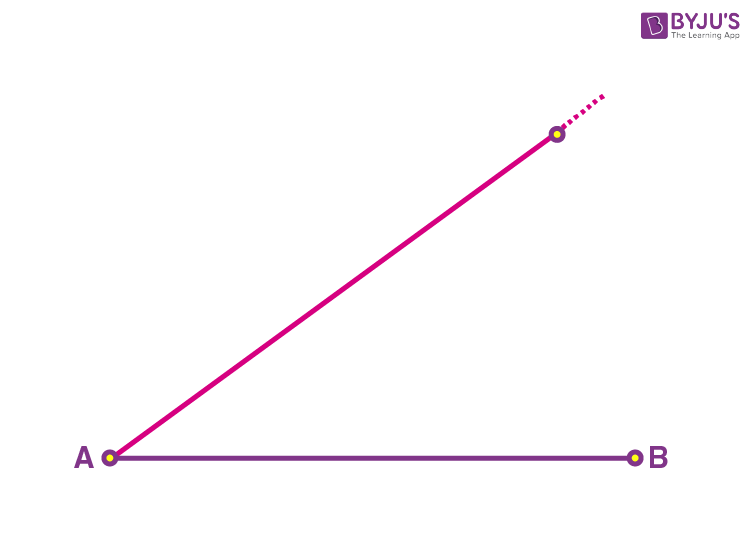Step 4: Again, place the centre of the protractor on point B and measure 70° [Use the outer reading]. Now, put a mark at 70° and name the intersection point as C.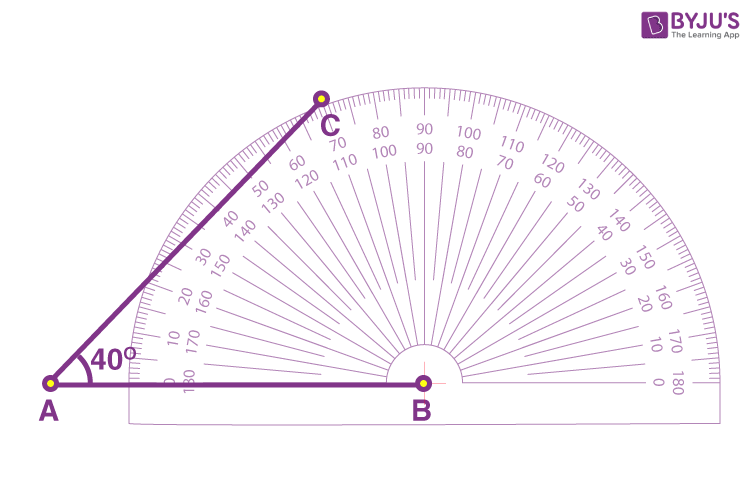Step 5: Now, draw a line by joining points B and C.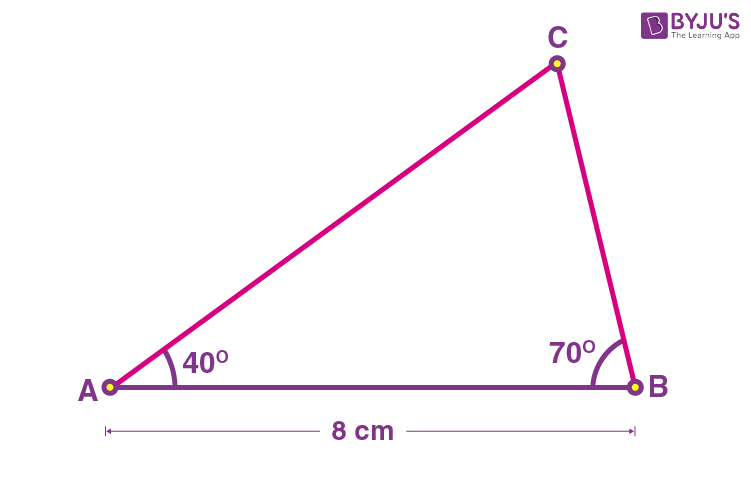Hence, ABC is the required triangle.

### Video Lesson on TrianglesStay tuned with BYJU’S – The Learning App and download the app today to learn all Maths-related concepts quickly by exploring more videos.